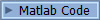(Nonparametric/Semiparametric Estimation)

KleinSpady.m implements the Klein-Spady estimator (Klein and Spady, 1993) for a semiparametric binary choice model of the form D = 1(X*beta + epsilon >= 0), where the distribution of epsilon is unknown and is estimated nonparametrically, i.e., by kernel regression or local linear regression. KleinSpady.m returns estimated coefficients, marginal effects, and the error density function evaluated at the index mean.

Ichimura.m implements the Ichimura estimator for a semiparametric regression model Y = g(X*b)+e, using kernel regression with the Quartic kernel. The Ichimura estimator can only identify beta up to location and scale, so the first coefficient is normalized to 1. [beta, margEff, deriv_g] = Ichimura (X,Y,h) returns coefficients, marginal effects, and the derivative of g function evaluated at the index mean, g'(Xbar*b). beta = Ichimura(X,Y) implements the Ichimura estimator, using the optimal bandwidth computed by Silverman's rule. beta = Ichimura(X,Y,h) implements the Ichimura estimator, using the user provided bandwidth, h.

ks.m implements multi-dimensional nonparametric regression, (local constant) kernel regression or local linear regression. It uses either user-provided  bandwidth or optimal bandwidth obtained by cross-validation. ks.m returns the fitted value of Y (Yhat) at each observation of X.

opt_h.m implements cross-validation and returns the optimal bandwidth for multi-dimensional nonparametric regression, i.e., kernel regression or local linear regression.

gmm.do does linear/nonlinear GMM estimation. The number of parameters and the moment functions need to be provided by users. This code also includes hree examples. The first is GMM estimation of a binary choice model. The related model has 5 parameters and 5 moment conditions. The second is GMM estimation of a sample selection model. The related model has 8 parameters and 8 moment conditions. The third example is GMM estimation of the return to schooling application in Lewble(2005). The related model has 19 parameters and 19 moment conditions.

simpbin0/ simpbin1/ simpbin2 estimate a binary choice model with possible endogenous regressors, given a very exogenous regressor (V) (Lewbel, 2005).

1. simpbin0 provides the simple binary choice estimator when the density of V is known.
2. simpbin1 provides the simple binary choice estimator assuming V is conditionally normal.
3. simpbin2 provides the simple binary choice estimator using a nonparametric sorted-data density for V model error.

simpsel1/simpsel2 estimate a selected sample model with possibly endogenous regressors, given a very exogenous regressor (V) in its selection indicator function (Lewbel, 2005).

1.simpsel1 provides a simple sample selection estimator assuming the selection model error is normal.
2.simpsel2 provides a simple sample selection estimator using a nonparametric sorted-data density of the selection model error.

1.sortedf1 is a command to calculate a nonparametric sorted-data density (Lewbel & Schennach, 2007). It is called by simpbin2 and simpsel2.
2.sortuniq1 is a command called by sortedf1. It sorts the data and keeps the unique values in ascending order.
3.zrnd is a command to round a near zero number away from zero.